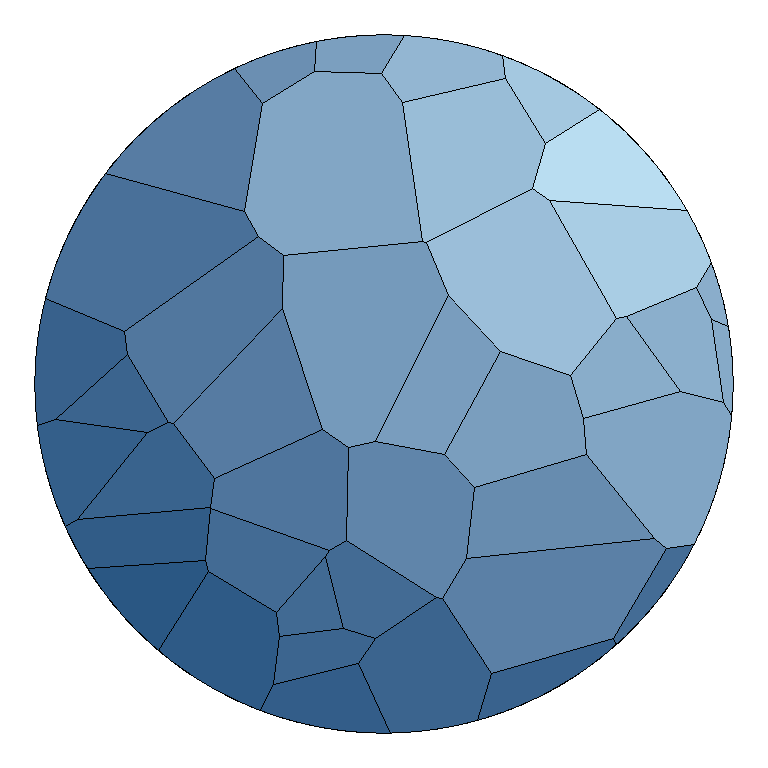# Voronoi diagram in ggplot2 with ggvoronoi

#### Author

Robert C. Garrett

## Sample data

The data set below contains three columns where the first are the values of the X-axis variable, the second the values of the Y-axis variable and the third the euclidean distance between the points and the center of the plot, which is the point (200, 200).

``````# Data
set.seed(1)
x <- sample(1:400, size = 100)
y <- sample(1:400, size = 100)
dist <- sqrt((x - 200) ^ 2 + (y - 200) ^ 2)

df <- data.frame(x, y, dist = dist)``````

## Voronoi diagram with `stat_voronoi`

The `stat_voronoi` function can be used to create a simple Voronoi diagram, passing the `x` and `y` variables to the `aes` function and using `stat_voronoi(geom = "path")`.

``````# install.packages("ggvoronoi")
# install.packages("ggplot2")
library(ggvoronoi)
library(ggplot2)

ggplot(df, aes(x, y)) +
stat_voronoi(geom = "path")``````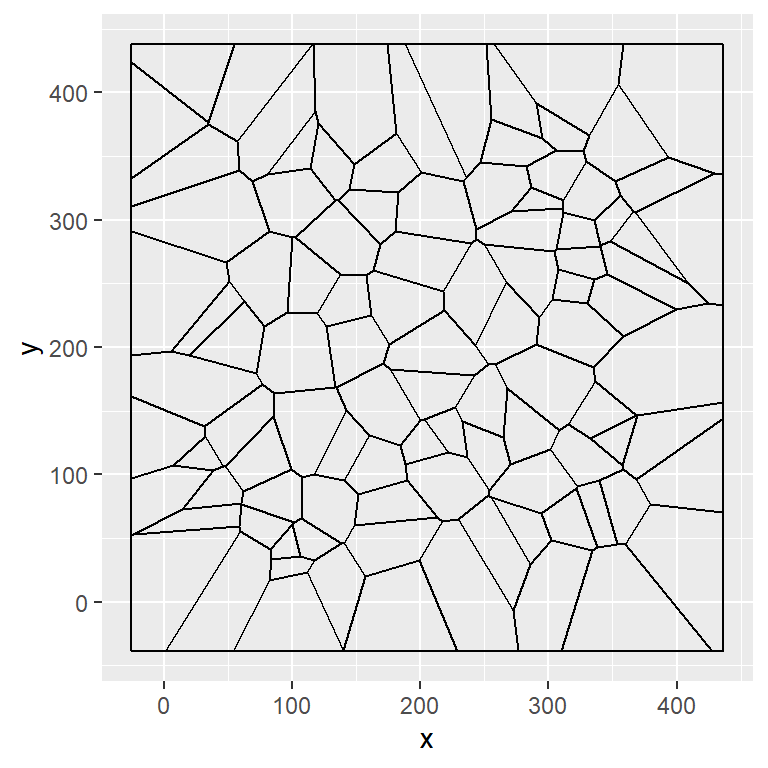Observations

You can also add the observations making use of `geom_point`.

``````# install.packages("ggvoronoi")
# install.packages("ggplot2")
library(ggvoronoi)
library(ggplot2)

ggplot(df, aes(x, y)) +
stat_voronoi(geom = "path") +
geom_point()``````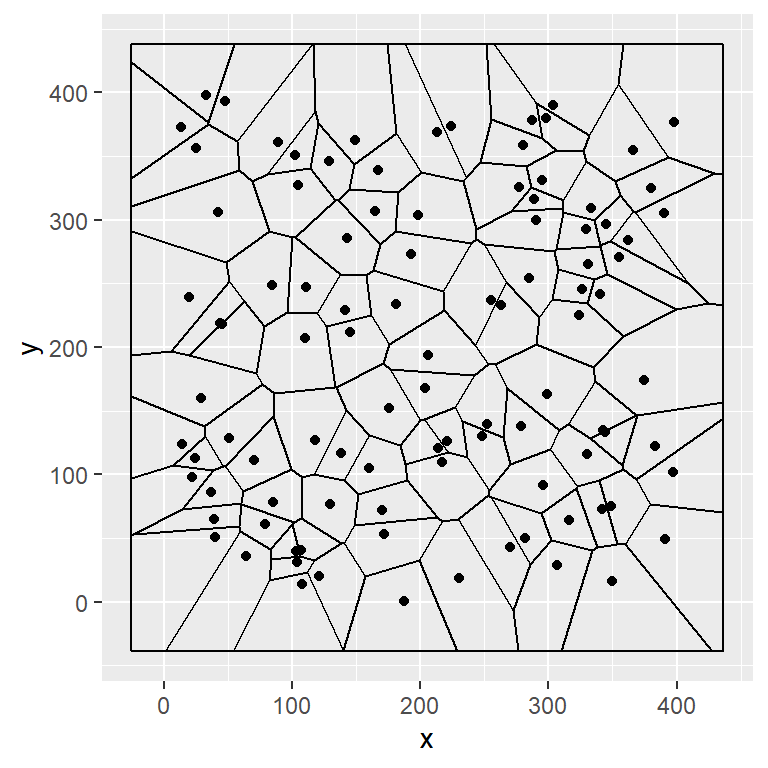Path style

Note that you can change the styling of the lines with the `color`, `lwd` and `linetype` arguments.

``````# install.packages("ggvoronoi")
# install.packages("ggplot2")
library(ggvoronoi)
library(ggplot2)

ggplot(df, aes(x, y)) +
stat_voronoi(geom = "path",
color = 4,      # Color of the lines
lwd = 0.7,      # Width of the lines
linetype = 1) + # Type of the lines
geom_point()``````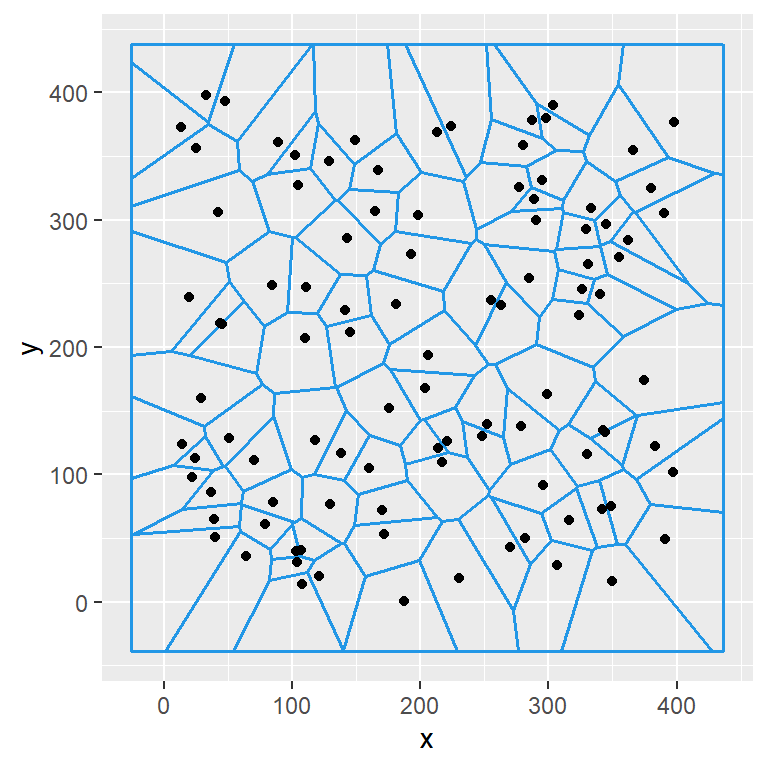## Voronoi heat map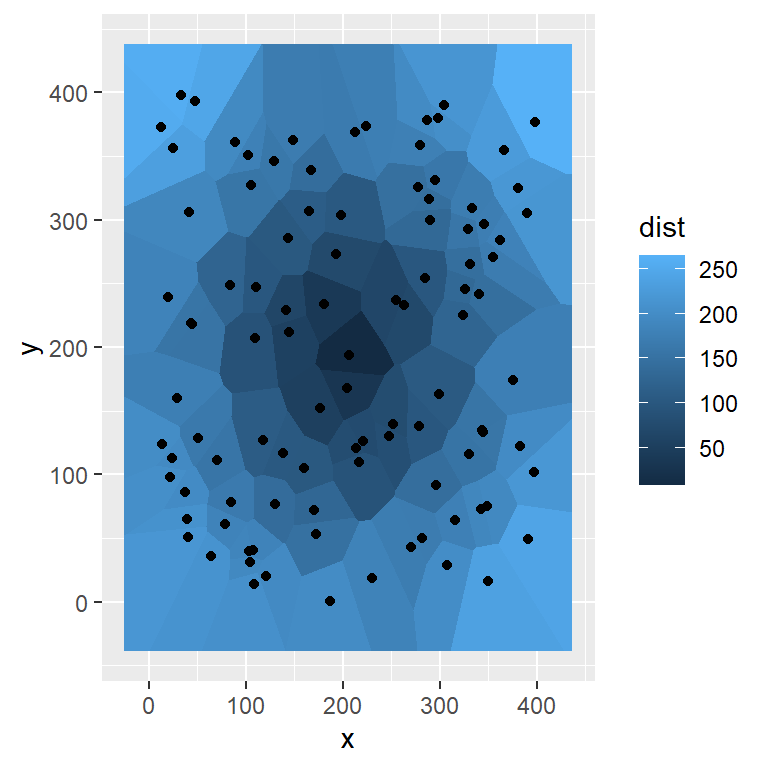You can pass a variable to the `fill` argument of the `aes` function to create a Voronoi heat map. For this purpose you will need to use the `geom_voronoi` function.

``````# install.packages("ggvoronoi")
# install.packages("ggplot2")
library(ggvoronoi)
library(ggplot2)

ggplot(df, aes(x, y, fill = dist)) +
geom_voronoi() +
geom_point()``````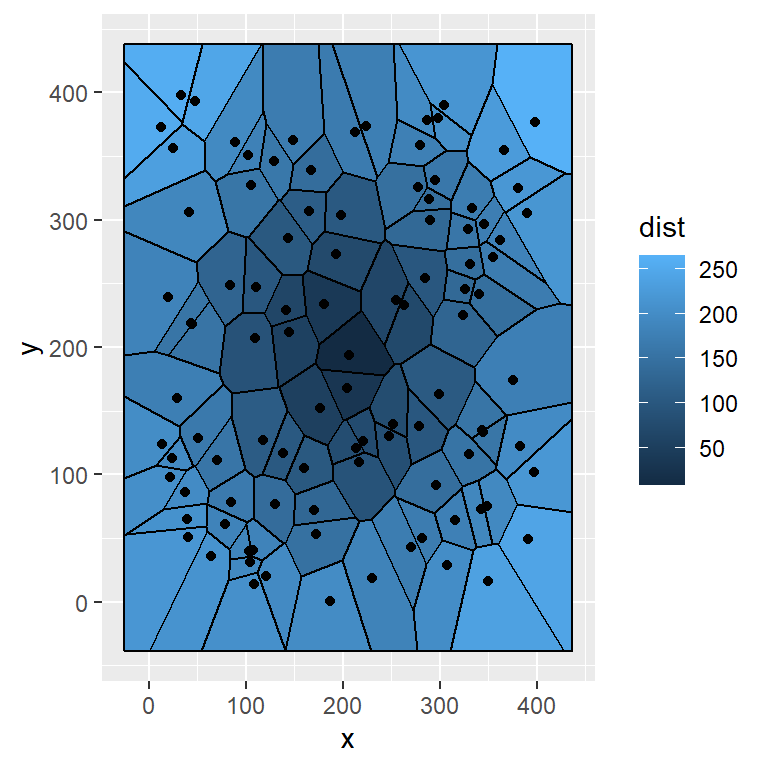The path of the diagram can be added passing `stat_voronoi(geom = "path")`.

``````# install.packages("ggvoronoi")
# install.packages("ggplot2")
library(ggvoronoi)
library(ggplot2)

ggplot(df, aes(x, y, fill = dist)) +
geom_voronoi() +
stat_voronoi(geom = "path") +
geom_point()``````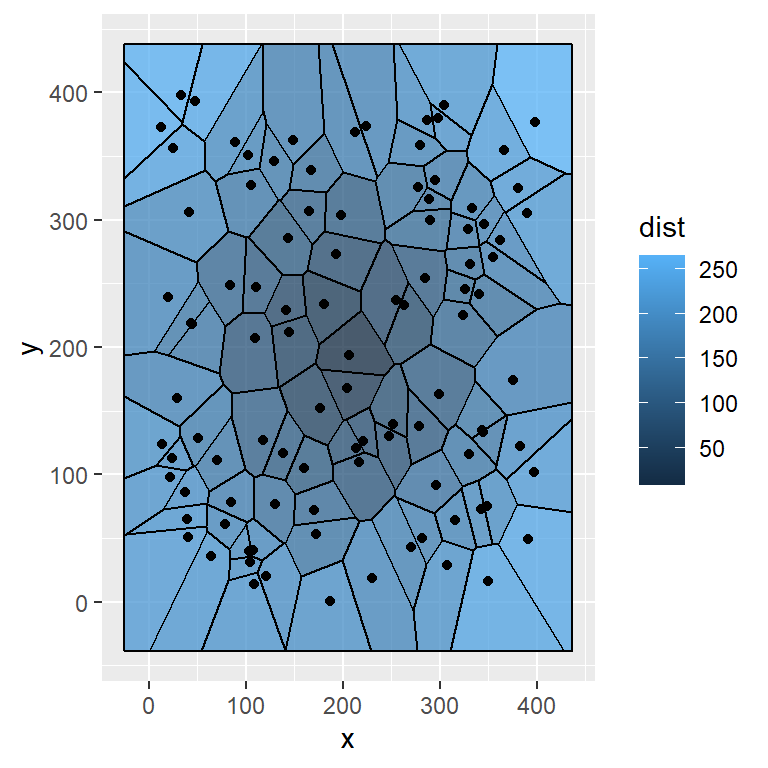The transparency of the color can be set with the `alpha` argument of the `geom_voronoi` function.

``````# install.packages("ggvoronoi")
# install.packages("ggplot2")
library(ggvoronoi)
library(ggplot2)

ggplot(df, aes(x, y, fill = dist)) +
geom_voronoi(alpha = 0.75) +
stat_voronoi(geom = "path") +
geom_point()``````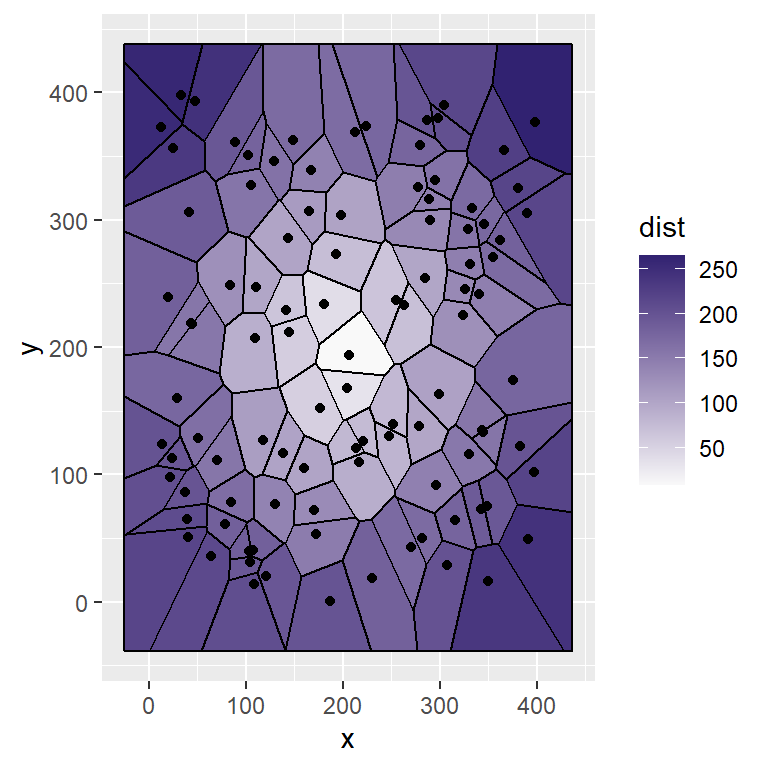In addition, if you want to change the color for the heat map you can use `scale_fill_gradient`, setting a low and high color value as in the example below.

``````# install.packages("ggvoronoi")
# install.packages("ggplot2")
library(ggvoronoi)
library(ggplot2)

ggplot(df, aes(x, y, fill = dist)) +
geom_voronoi() +
stat_voronoi(geom = "path") +
geom_point() +
high = "#312271")``````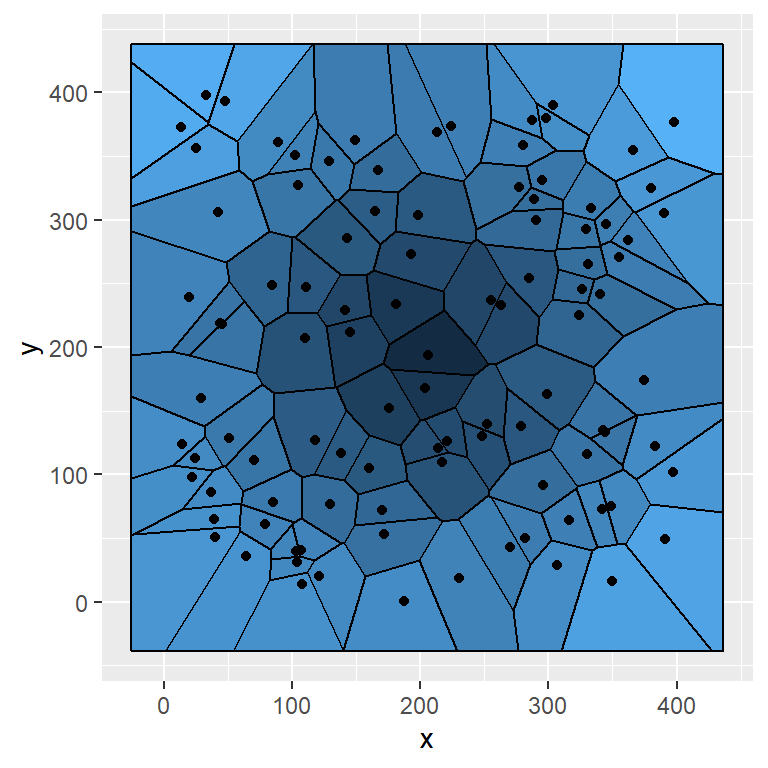Note that if you want to remove the legend you can set `legend.position = "none"` inside the `theme` function. You can also set other positions.

``````# install.packages("ggvoronoi")
# install.packages("ggplot2")
library(ggvoronoi)
library(ggplot2)

ggplot(df, aes(x, y, fill = dist)) +
geom_voronoi() +
stat_voronoi(geom = "path") +
geom_point() +
theme(legend.position = "none")``````

## Bounding box

You can set your own bounding box passing a data frame to the `outline` argument of the `geom_voronoi` function, being the first column the longitude, the second the latitude and the third a group column.

``````# install.packages("ggvoronoi")
# install.packages("ggplot2")
library(ggvoronoi)
library(ggplot2)

# Circle
s <- seq(0, 2 * pi, length.out = 3000)
circle <- data.frame(x = 120 * (1 + cos(s)),
y = 120 * (1 + sin(s)),
group = rep(1, 3000))

ggplot(df, aes(x, y, fill = dist)) +
geom_voronoi(outline = circle,
color = 1, size = 0.1) +